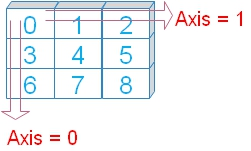# Numpy n dimensional Arrays ( ndarray )

NumPy (Numerical Python) is a popular open-source Python library used for numerical computing and data analysis.

NumPy is often used in combination with other Python libraries, such as Pandas, Matplotlib, and Scikit-learn, to create a complete data analysis ecosystem in Python.

## Some of the key features of NumPy :

1. Fast vectorized operations: NumPy allows you to perform operations on entire arrays rather than looping through each element, which can be much faster.
2. Broadcasting: NumPy allows you to perform operations between arrays with different shapes and sizes, which makes it easy to perform operations on data that is not the same shape.
3. Linear algebra: NumPy provides a suite of functions for performing linear algebra operations such as matrix multiplication, eigenvalues, and eigenvectors.
4. Random number generation: NumPy includes tools for generating random numbers, which are useful for simulations and statistical analysis.Numpy is used scientific computing with Python.
We can create powerful multidimensional arrays using Numpy.
Numpy requires all elements are to be of same data type. ( This is the main difference with Pandas which can accommodate different data types. )

## Installing Numpy

Use in your command prompt or the environment you are using . `pip install numpy` After installation you can add this line to import Numpy inside Python program.
``import numpy as np``
Getting the Numpy version installed in your system.
``````import numpy as np
print("Numpy Version : ",np.__version__)``````

## Creating Numpy Array

``````import numpy as np
npr=np.array([4,5,9])
print(npr) # [4,5,9]

l1=[3,2,8] # List
npr=np.array(l1) # Using List to create np array
print(npr) # [3,2,8]

t1=(8,12,1) # Tuple
npr=np.array(t1) # from tuple to np array
print(npr) # [ 8 12  1]``````

Numpy installation and creating array at Colab platform using List Tuple and checking the version

## Numpy

 NumPy Array Scientific computing with Python append Adding Data to array at the end insert Adding Data to array at the given index position dtype Data types of Numpy array Array Methods Methods to get result ( output ) using NumPy array_split() break the array to get sub-arrays bincount frequency of occurrence of elements where Return elements depending on condition check shape Return shape of the array reshape Change the array shape and dimension

## Creating array

 create Creating array using Numpy eye array with ones and zeros empty array without initializing entries empty_like array of same shape and type full Array filled with input value ones array filled with ones arange creating array of fixed steps linspace creating array of fixed number zeros Aray filled with zeros
 Exercise Creating different types of array

 random.randit Random integers with lower and upper limits and size random.rand Uniform distribution [0,1] of random numbers of given shape & population random.randn Normal distribution of random numbers of given shape & population random_sample Continuous uniform distribution over an interval

 sum() Sum of elements, or along the axis mean() Mean value of elements, or along the axis max() Maximum value among elements, or along the axis min() Minimum value among elements, or along the axis std() Standard deviation along the axis
 Exercise a Basics of Creating Arrays
 math functions Numpy math functions
Get the list of all functions of Pandas. ( Use dir() )
``````import numpy as np
print(len(dir(np))) # 622``````
List of functions
``````for i in dir(np):
print(i)``````

Subscribe to our YouTube Channel here

## Subscribe

* indicates required
Subscribe to plus2netplus2net.com